I recently took a Environmental Engineering graduate course about contaminant transport. Part of the course involved finite difference analysis and modelling so I thought I’d do a simple visualization with Processing to see the equations in action. The coding part was pretty simple but it was interesting to see how I could apply Processing to visualize a contaminant plume.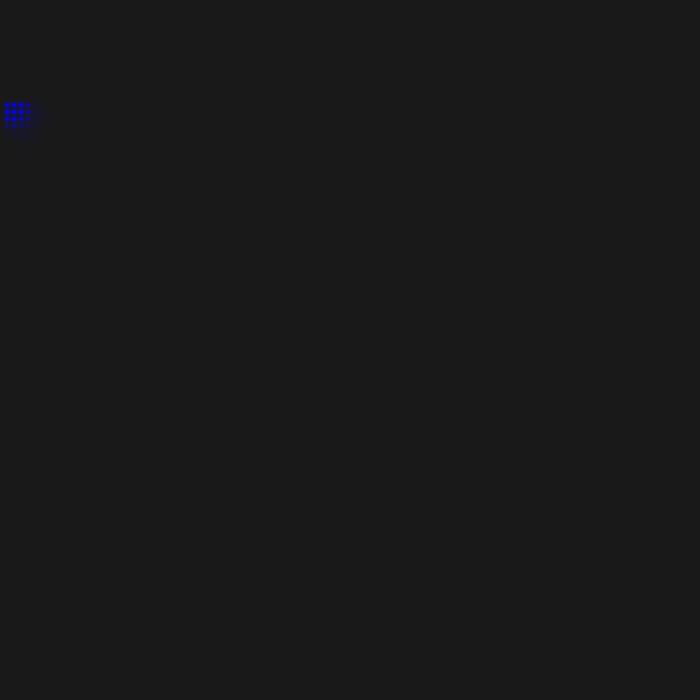I’ll get into some basic contaminant transport equations but before I do that here are some terms and definitions:

Advection (v): the transport of a contaminant due to velocity and concentration

Dispersion (D): the transport of a contaminant due to changes in velocity leading to mixing and dilution.

Reaction Kinetics (K): refers to the rate of reaction of a contaminant as it propagates through a medium. For this model we’re assuming pseudo first order reactions, where the the reaction rate is dependent on a single component.

Given the terms above we can determine the change in concentration (C) at a given time based on advection (v), dispersion (D) and reaction (K):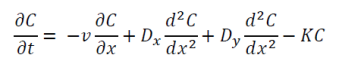We can then use the Taylor series finite difference method to obtain an explicit solution to the partial differential equation and determine the concentration at each point at the next time step: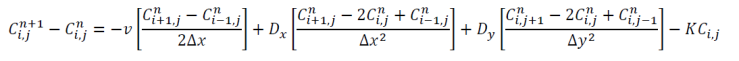Rearranging will give us: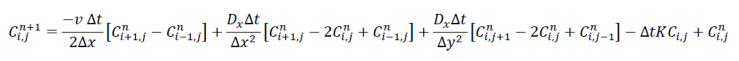To simplify the equation (and for simple testing of the model), we’ll assume values for a, b, c & d: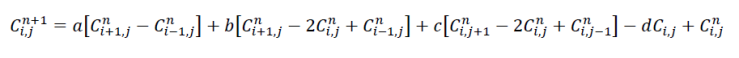Since I’m using the finite different method it wasn’t the most efficient way to visually model the flow of the contaminant. It would be too intensive to calculate the values for the each pixel in the canvas in Processing so I created a sort of dot matrix with circles. The effect is reasonable and does manage to visually convey the plume although somewhat laggy. I’m interested to see how you could model advection-dispersion using some sort of particle system simulation based on something like this.

Here is the code

Reference: ENEN 627 Contaminant Transport, University of Calgary, J. Hollman (2017).